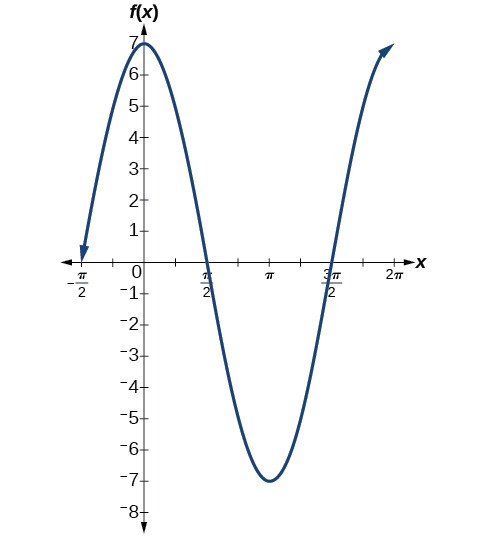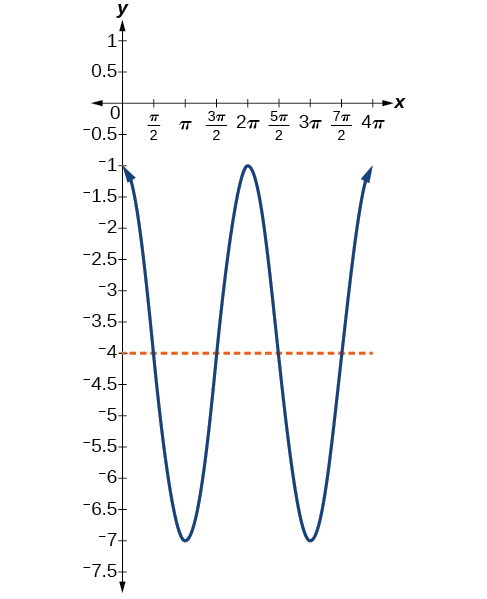8.1 Graphs of the sine and cosine functions  (Page 6/13)

 Page 6 / 13

Identifying the properties of a sinusoidal function

Given $\text{\hspace{0.17em}}y=-2\mathrm{cos}\left(\frac{\pi }{2}x+\pi \right)+3,\text{\hspace{0.17em}}$ determine the amplitude, period, phase shift, and horizontal shift. Then graph the function.

Begin by comparing the equation to the general form and use the steps outlined in [link] .

$y=A\mathrm{cos}\left(Bx-C\right)+D$
• Step 1. The function is already written in general form.
• Step 2. Since $\text{\hspace{0.17em}}A=-2,\text{\hspace{0.17em}}$ the amplitude is $\text{\hspace{0.17em}}|A|=2.$
• Step 3. $\text{\hspace{0.17em}}|B|=\frac{\pi }{2},\text{\hspace{0.17em}}$ so the period is $\text{\hspace{0.17em}}P=\frac{2\pi }{|B|}=\frac{2\pi }{\frac{\pi }{2}}=2\pi \cdot \frac{2}{\pi }=4.\text{\hspace{0.17em}}$ The period is 4.
• Step 4. $\text{\hspace{0.17em}}C=-\pi ,$ so we calculate the phase shift as $\text{\hspace{0.17em}}\frac{C}{B}=\frac{-\pi ,}{\frac{\pi }{2}}=-\pi \cdot \frac{2}{\pi }=-2.\text{\hspace{0.17em}}$ The phase shift is $\text{\hspace{0.17em}}-2.$
• Step 5. $D=3,$ so the midline is $\text{\hspace{0.17em}}y=3,$ and the vertical shift is up 3.

Since $\text{\hspace{0.17em}}A\text{\hspace{0.17em}}$ is negative, the graph of the cosine function has been reflected about the x -axis.

[link] shows one cycle of the graph of the function.

Using transformations of sine and cosine functions

We can use the transformations of sine and cosine functions in numerous applications. As mentioned at the beginning of the chapter, circular motion can be modeled using either the sine or cosine function    .

Finding the vertical component of circular motion

A point rotates around a circle of radius 3 centered at the origin. Sketch a graph of the y -coordinate of the point as a function of the angle of rotation.

Recall that, for a point on a circle of radius r , the y -coordinate of the point is $\text{\hspace{0.17em}}y=r\text{\hspace{0.17em}}\mathrm{sin}\left(x\right),\text{\hspace{0.17em}}$ so in this case, we get the equation $\text{\hspace{0.17em}}y\left(x\right)=3\text{\hspace{0.17em}}\mathrm{sin}\left(x\right).\text{\hspace{0.17em}}$ The constant 3 causes a vertical stretch of the y -values of the function by a factor of 3, which we can see in the graph in [link] .

What is the amplitude of the function $\text{\hspace{0.17em}}f\left(x\right)=7\mathrm{cos}\left(x\right)?\text{\hspace{0.17em}}$ Sketch a graph of this function.

7Finding the vertical component of circular motion

A circle with radius 3 ft is mounted with its center 4 ft off the ground. The point closest to the ground is labeled P , as shown in [link] . Sketch a graph of the height above the ground of the point $\text{\hspace{0.17em}}P\text{\hspace{0.17em}}$ as the circle is rotated; then find a function that gives the height in terms of the angle of rotation.

Sketching the height, we note that it will start 1 ft above the ground, then increase up to 7 ft above the ground, and continue to oscillate 3 ft above and below the center value of 4 ft, as shown in [link] .

Although we could use a transformation of either the sine or cosine function, we start by looking for characteristics that would make one function easier to use than the other. Let’s use a cosine function because it starts at the highest or lowest value, while a sine function    starts at the middle value. A standard cosine starts at the highest value, and this graph starts at the lowest value, so we need to incorporate a vertical reflection.

Second, we see that the graph oscillates 3 above and below the center, while a basic cosine has an amplitude of 1, so this graph has been vertically stretched by 3, as in the last example.

Finally, to move the center of the circle up to a height of 4, the graph has been vertically shifted up by 4. Putting these transformations together, we find that

$y=-3\mathrm{cos}\left(x\right)+4$

A weight is attached to a spring that is then hung from a board, as shown in [link] . As the spring oscillates up and down, the position $\text{\hspace{0.17em}}y\text{\hspace{0.17em}}$ of the weight relative to the board ranges from $\text{\hspace{0.17em}}–1\text{\hspace{0.17em}}$ in. (at time $\text{\hspace{0.17em}}x=0\right)\text{\hspace{0.17em}}$ to $\text{\hspace{0.17em}}–7\text{\hspace{0.17em}}$ in. (at time $\text{\hspace{0.17em}}x=\pi \right)\text{\hspace{0.17em}}$ below the board. Assume the position of $\text{\hspace{0.17em}}y\text{\hspace{0.17em}}$ is given as a sinusoidal function of $\text{\hspace{0.17em}}x.\text{\hspace{0.17em}}$ Sketch a graph of the function, and then find a cosine function that gives the position $\text{\hspace{0.17em}}y\text{\hspace{0.17em}}$ in terms of $\text{\hspace{0.17em}}x.$

$y=3\mathrm{cos}\left(x\right)-4$A laser rangefinder is locked on a comet approaching Earth. The distance g(x), in kilometers, of the comet after x days, for x in the interval 0 to 30 days, is given by g(x)=250,000csc(π30x). Graph g(x) on the interval [0, 35]. Evaluate g(5)  and interpret the information. What is the minimum distance between the comet and Earth? When does this occur? To which constant in the equation does this correspond? Find and discuss the meaning of any vertical asymptotes.
The sequence is {1,-1,1-1.....} has
how can we solve this problem
Sin(A+B) = sinBcosA+cosBsinA
Prove it
Eseka
Eseka
hi
Joel
June needs 45 gallons of punch. 2 different coolers. Bigger cooler is 5 times as large as smaller cooler. How many gallons in each cooler?
7.5 and 37.5
Nando
find the sum of 28th term of the AP 3+10+17+---------
I think you should say "28 terms" instead of "28th term"
Vedant
the 28th term is 175
Nando
192
Kenneth
if sequence sn is a such that sn>0 for all n and lim sn=0than prove that lim (s1 s2............ sn) ke hole power n =n
write down the polynomial function with root 1/3,2,-3 with solution
if A and B are subspaces of V prove that (A+B)/B=A/(A-B)
write down the value of each of the following in surd form a)cos(-65°) b)sin(-180°)c)tan(225°)d)tan(135°)
Prove that (sinA/1-cosA - 1-cosA/sinA) (cosA/1-sinA - 1-sinA/cosA) = 4
what is the answer to dividing negative index
In a triangle ABC prove that. (b+c)cosA+(c+a)cosB+(a+b)cisC=a+b+c.
give me the waec 2019 questionsByBy